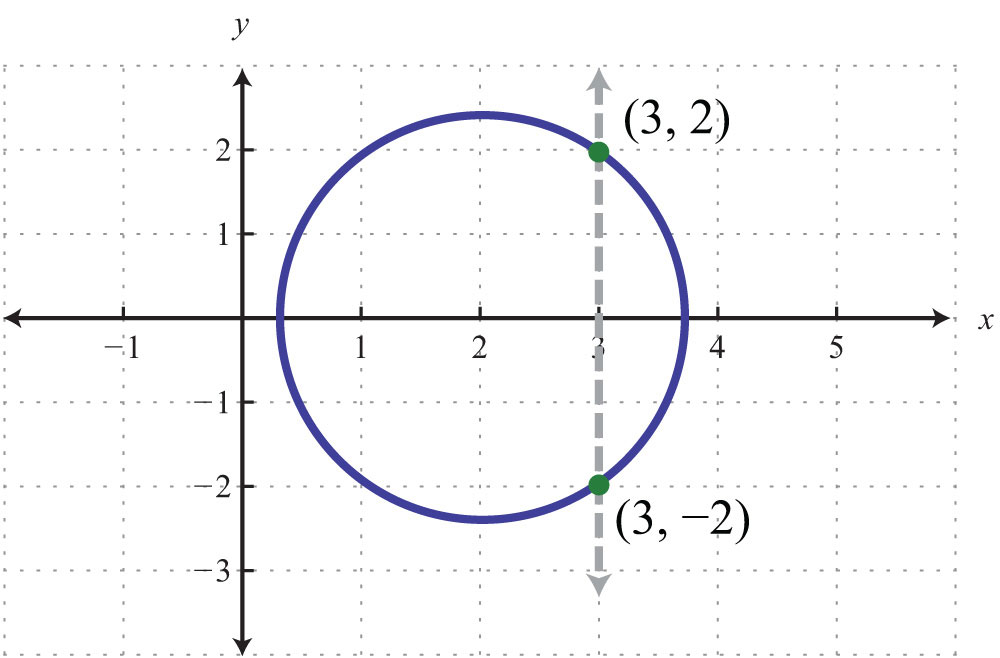Graph
Mapping
Table
Points
Evaluate the function to find y
100yes
100yes
100yes
100
(0, 0), (1, 1), (1, -1), (2, 2), (2, -2)
no
100
y = 10x; when x = − 3
30
200no
200yes
200no
200
(4, 2), (5, 1), (6, 0), (7, -1), (8, -2)
no
200
y = 6 − 2x; when x = 11
y=-16
300no
300no
300no
300
(-2, 2), (-1, 1), (0, 0), (1, 1), (2, 2)
yes
300
y = -4x + 5; when x =1/2
y=3
400Which are functions?
A and B
400yes
400yes
400
{(1,2), (2,4), (3,5)}
yes
400
Find x when: y = 9 − 7x; y = 37
x=-4
500
A graph is a function if it passes the _____________ line test
vertical
500yes
500no
500
(–3, 5), (–2, 5), (1, 5), (0, 5), (1, 5), (2, 5)
no
500
y = 1/4x −7; y = 2
x=36
Click to zoom